# Gear Basics Part 2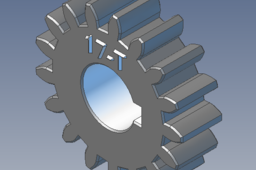We will start off this second gear design tutorial by looking at a few design factors we need to consider when designing a serviceable gear train.

1. ### Step 1: Overview

The method I have used here to design gears is based on making use of standards and codes. A large part of this document is based on a textbook “Applied Mechanical Design” by A. K. Hosking & M. R. Harris 1st and 2nd edition, chapter 3 Gear Design. I have used many charts and diagrams from this book. The design process used is based on standards and codes, the standards used in this design process are the British Standard BS 436 and the more modern ISO 54 and AS 2938. This method formalizes the design process and puts everyone on the same page when designing equipment that requires certification to be put into service. The modern approach often is to ignore the standards based method and go to all the new toys, FEM, and such, but FEM depends a large part on the knowledge and skill of the operator, the right answer is only obtained when the analysis is done correctly, this only happens if the operator understands the real forces and stresses involved. With the standards method if you follow it correctly you will get a result that will work, and if your equipment requires certification the certifying body will understand your results.

2. ### Step 2:

. Suitable gear ratio – for simple spur and helical gears the maximum ratio we should design for our gear set is 10 : 1, often in practice this is kept to around 3 : 1. The reasons for this is due to gears being used to change a lower RPM into a higher RPM, they are also used to change a lower torque to a higher torque . in the torque case with a 10 : 1 ratio, a tooth on the higher torque gear has to handle a loading 10 times more than the lower torque gear. Designing our gears for strength is easier at ratio’s less than 10 : 1, and simpler at a 3 : 1 ratio. Compound gear trains and other gearing systems come into use when larger ratio’s are required. In these tutorials we are only looking at simple gear trains.

2. As with many mechanical components we design, we take the actual loads involved and apply a “service factor”. We multiply the load applied by a factor to compensate for any variation caused by the nature of the driven machine and the driving machine. The following table taken from AS 2938 shows typical service factors to use in some cases.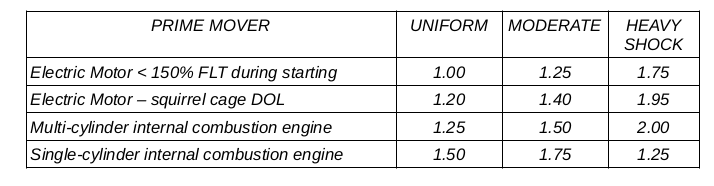1. Material – sometimes the pinion being the smaller component is made from a higher grade of material than the gear, though often as it is more convenient we make the pinion and gear from the same material. When using higher grades of steel we will cut the gear in the annealed condition then harden and temper to achieve the required strength and hardness. Gears that have been hardened need often to be ground afterwards with an allowance on the teeth and bore for this process. A protuberance is required so that the tooth can be
2. correctly ground ( see diagram 1 ).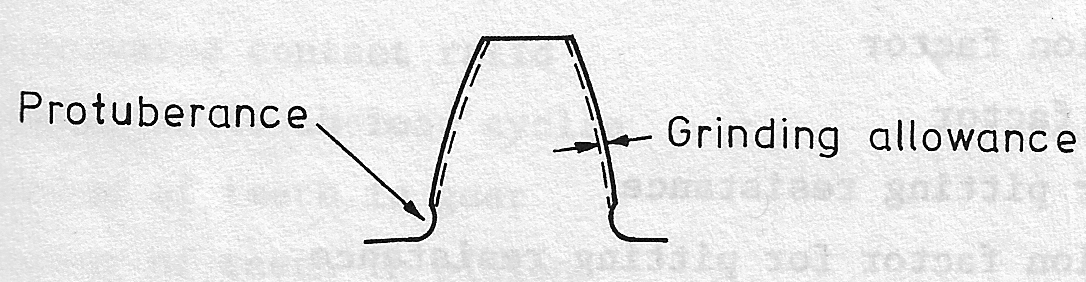3. ### Step 3:

A quick review of formula from Gear Basics Part 1.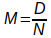where M = the gear module, D = operating pitch diameter of the gear, N = the number of teeth the gear has.

D (pitch dia. gear ) = N( no. of teeth gear) x M (tooth module) – this is for the driven gear

d (pitch dia. Pinion) = n (no. of teeth pinion) x M (tooth module) – for the pinion gear we use the same basic formula but change to lower case to indicate this is pinion calculation

calculate center distance between gears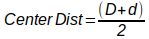We will now add formula used to calculate blank diameter required to cut gear from

Bdia (blank diameter gear) = (N + 2) x M and for pinion basically the same but lower case

bdia (blank diameter pinion) = (n + 2) x M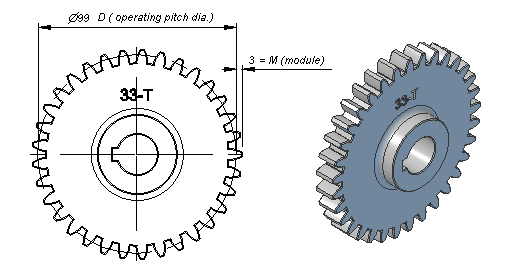Example : Gear

Bdia= (N + 2)x M

= (33 + 2) x 3

= 105mm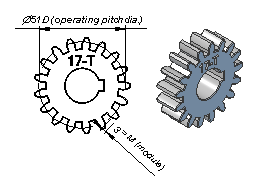Pinion

bdia = (n + 2) x M

= (17 + 2) x 3

= 57mm

4. ### Step 4: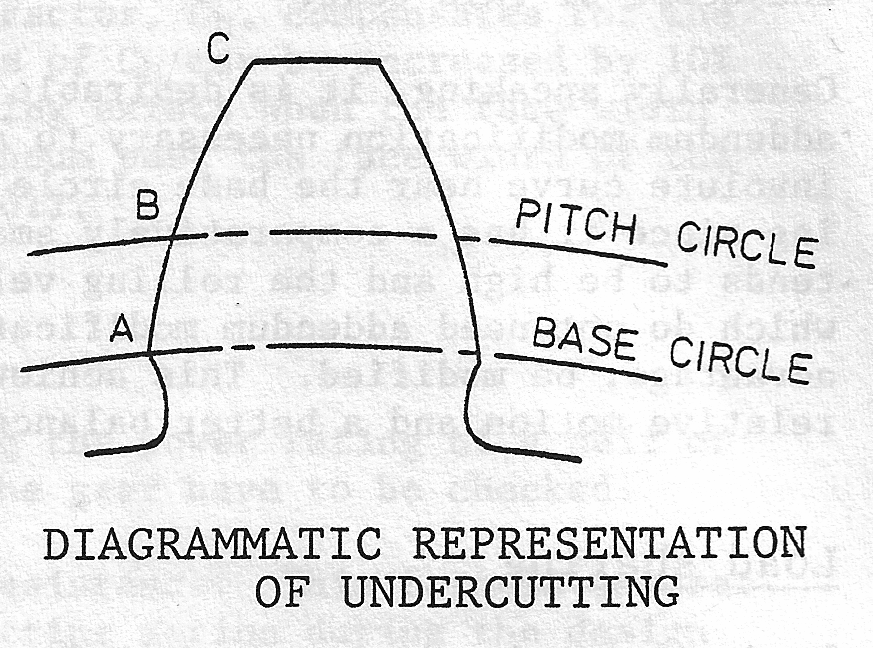Factors to Consider for Pinion Design

As previously mentioned in Gear Basics Part 1, with a 20 deg pressure angle undercutting will occur if the pinion has less than 17 teeth. Undercutting reduces the strength of the pinion teeth and the teeth mating will be poorer. For good proportions we make the gear face width between 8 and 12 times the tooth module. The pinion face width is normally about wider than the gear by between 4mm to 10mm depending on its size, being mainly for appearance and to give a wider tolerance for assembly, the gear should always have full face contact.

When we need to use a gear with less than 17 teeth we can avoid some of the problems of undercutting by applying positive addendum modification ( correction ). A positive correction means that we make the gear outside diameter and the root diameter are made slightly larger, and a negative correction, we make these slightly smaller. The exact values we use is more of a specialist function so it will not be covered in this document.

To be able to work through gears, and be capable of being able to design gear-sets that will work in practice, requires a good understanding of power, torque along with basic rotational dynamics. The function of gear trains in our designed machines, is to both change rotational speed (rpm) and to convert the torque available from the prime mover to the torque required fro the function of our machine. It is important to be familiar with the formula’s, and calculations that apply to rotating machines. I have includes an appendix at the end of this document that runs through the basic power, rpm and torque as it applies to rotating machines.

5. ### Step 5: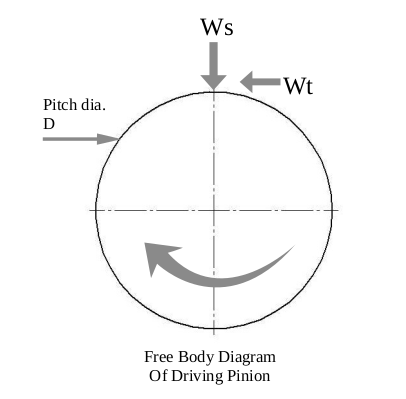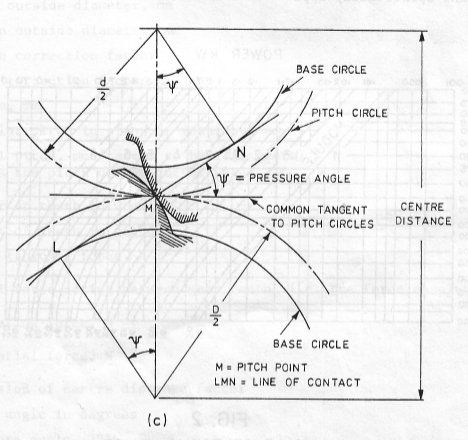Diagram 3 and 4 is the free body diagram for a gear that is the driving gear in a pair of gears.

The driving force Wn is the force acting between the surfaces ( line L – N diagram 4 ) this force can be replaced by components Wt and Ws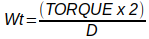The radial ( separation force ), Ws produces a compression force on the tooth and may be used with Wt for shaft design and bearing calculations. The tangential component, Wt causes bending stresses on the tooth and is used as a basis for the design of the tooth.

6. ### Step 6: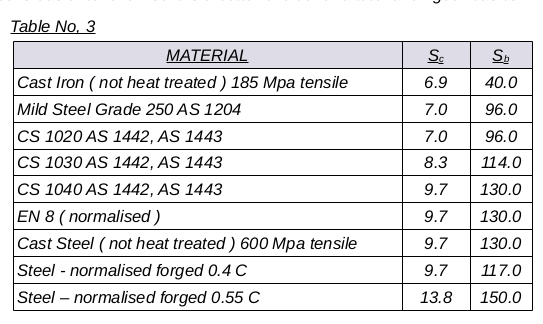Some of the common materials used in gears and the factors we use are listed in Table No. 3. This table in this publication lists only a few common materials that we will use in our example calculations in this guide. This table comes from BS 436. The calculation method also comes from this standard. This is the method used in the textbook “Applied Mechanical Design” by Hosking and Harris, the first edition. In the second edition of this book the chapter on gears instead of basing the gear design procedure from BS 436, uses SO Standard 54 and AS 2938 as a basis for gear design. I am using the older BS 436 as the last time, about 1997 when I last designed a gear train that was part of a wood chip loading system I only had the first edition of this book. The BS 436 method is easier to follow so it is a better choice for a tutorial on gear basics.

Rating Formula

We will now look at two formulae that we can use to rate the power handling capacity of a pair of gears. We check both the gear and the pinion.

The first formula looks at the pitting resistance of gears; This is to look at the gear’s resistance to failure due to pitting action over the design life of the gears. The formula, from BS 436 we apply to check a gear for resistance to failure due to pitting is :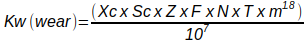where

• Xc is from chart 11 in BS 436 reproduced in pdf version
• Sc is the surface stress factor from Table No. 3.
• Z ( zone factor ) from chart 8 in BS 436 reproduced in pdf version.
• F = face width in mm.
• T = number of teeth.
• m = module in mm.
• N = rpm ( rev/min )

Example – Design for Pitting Resistance : Determine the face width required for the following spur gears

Power to transmit : 1.5 KW

Pinion : 32 teeth, 22 R.P.M, 0.4 % normalized steel

Gear : 44 teeth, 16 R.P.M, cast steel

4 hours per day operating time.

We have selected a gear module m of 6. This set of gears is slow speed. The chart bellow from BS 436 helps us to select module 6. We will use this in the calculation and the results will confirm if this was a good choice.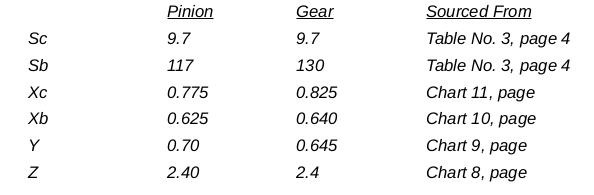Calculation Pinion for Wear

As our objective is to determine a face width acceptable for this gear we transpose our formula to solve for F and place the values for the pinion into this formula :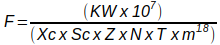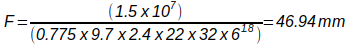Calculation Gear for Wear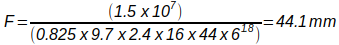We will now look at the calculation for gear strength. The formula, from BS 436 we apply to check a gear strength is :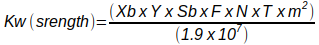where

• Xb is from chart 10 BS 436 reproduced on page 8
• Y is strength factor from chart 9 BS 436 reproduced on page 8
• Sb is the bending stress factor from Table No. 2
• All other definitions are listed previously in calc. For for wear

We will take the example for wear and now apply the formula for strength :

Pinion Strength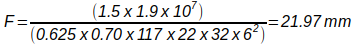Gear Strength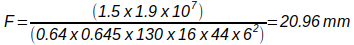As can be seen from the results of these calculation the pinion for wear calculation gives us the largest required face width. We would probably make the pinion about 50 mm wide and the gear about 45 mm wide.